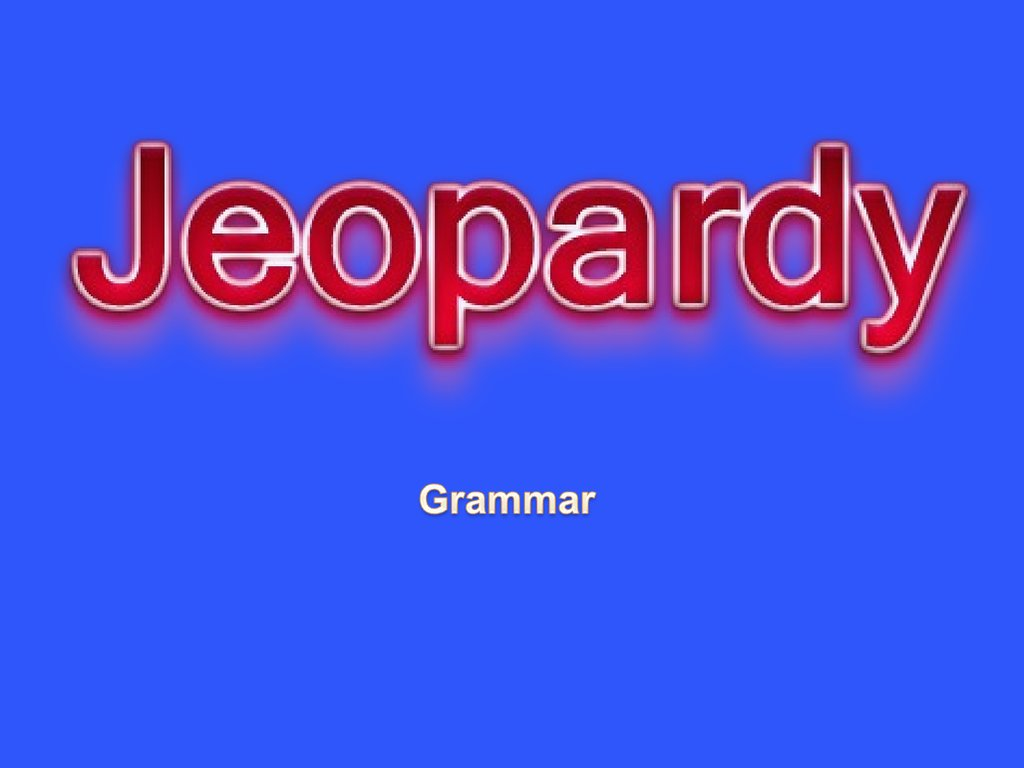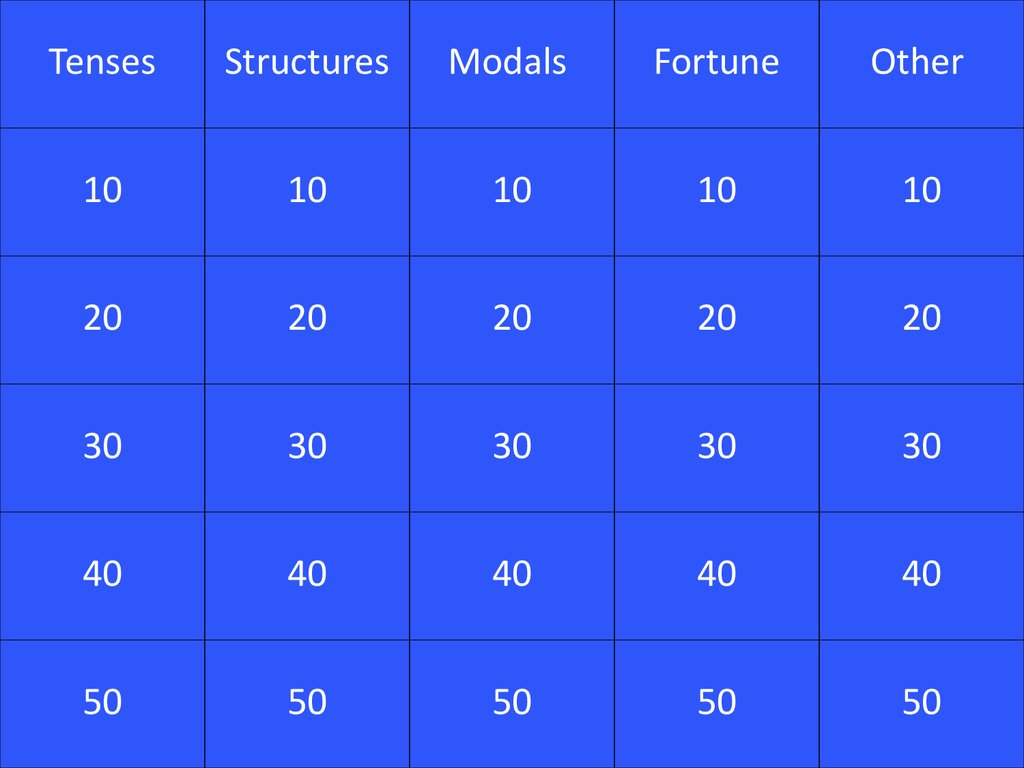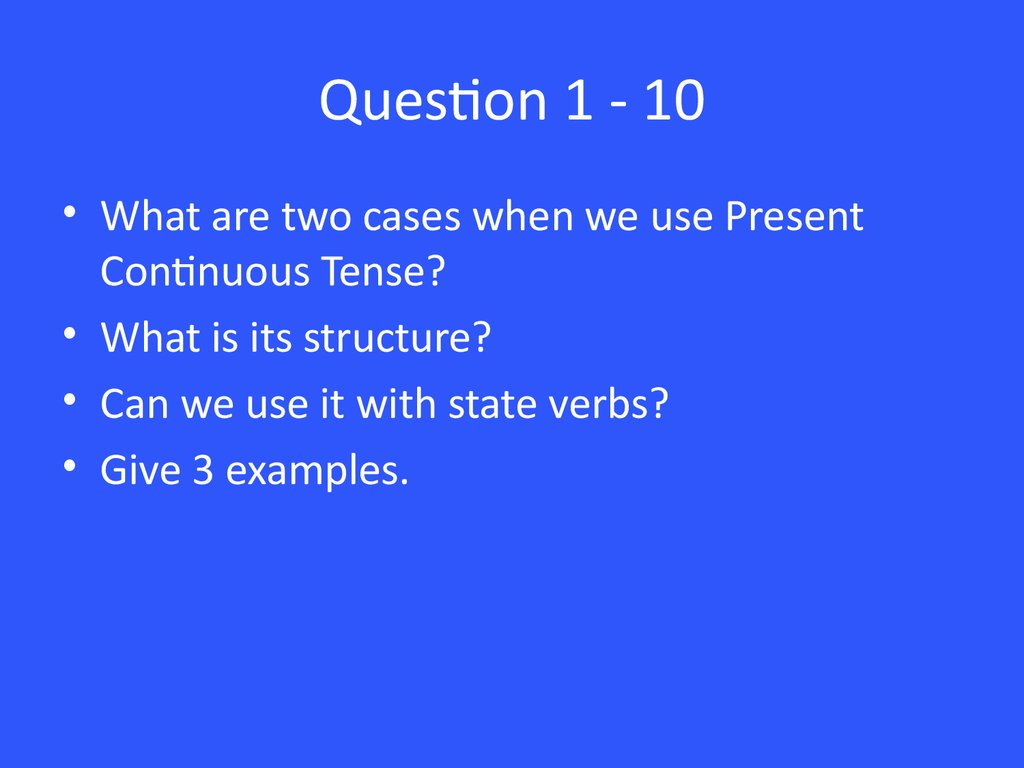# Jeopardy

Tenses
Structures
Modals
Fortune
Other
10
10
10
10
10
20
20
20
20
20
30
30
30
30
30
40
40
40
40
40
50
50
50
50
50

## 3. Question 1 - 10

• What are two cases when we use Present
Continuous Tense?
• What is its structure?
• Can we use it with state verbs?
• Give 3 examples.

## 5. Question 1 - 20

• When do we use Past Simple?
• What is its structure?
• Say the second form of the following verbs:
ride, teach, understand, catch.
• Give 3 examples of Past Simple sentences.

## 7. Question 1 - 30

When do we use Present Perfect Simple?
What is its structure?
Give 3 examples using for and since.
What is the meaning of just, yet and already?

## 9. Question 1 - 40

• When Past Perfect tense is used?
• What is its structure?

## 11. Question 1 - 50

• How do we use Past Continuous and Past
Simple in the sentence?
• What do when and while mean in such
sentences?

## 15. Question 2 - 20

• What structure do we use to speak about past
habits, that are not true now?
• Give 3 examples.

## 17. Question 2 - 30

• Make a sentence where a verb has two
objects, explain the grammar.

## 19. Question 2 - 40

When do we use “to be going to” structure?
How does it change depending on a person?
What time expressions are often used with it?
What is the difference between will and to be
going to?
• Give three examples.

## 21. Question 2 - 50

• How do we make comparative adjectives?
• What structures do we use to make
comparison?
• How do we make superlative adjectives?
• What are irregular adjectives? Give examples.

## 22. Answer 2 – 50

One syllable
Comparative
Superlative
Vowel + consonant = double consonant: big – bigger – the biggest
Two or more syllables
BUT: adjective with two or more syllables ending in –y changes
according to the rules of one syllable adjective: happy – happier –
the happiest.

## 23. Question 3 - 10

• What modals do we use to speak about
ability?
• Give examples.

## 25. Question 3 - 20

• What modal verbs do we use to make
• Give 3 examples.

## 27. Question 3 - 30

• What modal verb is used to talk about past
habits?
• What is the difference between would and
used to?

## 29. Question 3 - 40

• Which modal verbs do we use for permission
and obligation?
• Do we need an auxiliary verb when making
question and negative form with modals?
• Give 3 examples with different modals.

## 33. Question 4 - 10

• When do we use the following articles: a / an,
the, no article.
• Give an example for each rule.

## 35. Question 4 - 20

• What adverb of manner do you know?
• Are there any irregular verbs?
• What are the rules of forming adverbs of
manner?
• Give examples using each adverb.

## 37. Question 4 - 30

• What is reported speech?
• How do tenses change in the reported
speech?
• How do we usually start the reported speech?
• Give examples.

## 45. Question 5 - 20

• What is Present Simple Passive?
• What is its structure?
• Give 2 examples.
• What is Past Simple Passive?
• What is its structure?
• Give 2 examples.

## 47. Question 5 - 30

• What are the 2nd conditionals?
• Give 2 examples.

## 49. Question 5 - 40

• What are the 1st conditionals?
• Give 3 examples.

## 51. Question 5 - 50

• What are countable and uncountable nouns?
• What articles or words can we use before
them?
• When do we use too, too much / many and
(not) enough?
• Give examples.
• After which verbs do we use gerunds and
infinitives?